Email us to get an instant 20% discount on highly effective K-12 Math & English kwizNET Programs!

#### Online Quiz (WorksheetABCD)

Questions Per Quiz = 2 4 6 8 10

### Grade 3 - Mathematics8.16 Solids Shapes (Pyramids)

 A pyramid is any three-dimensional polyhedron where the faces other than the base are triangular and meet at one point, called the vertex or apex. The base of a pyramid can be any polygon: a square, or a triangle, leading to four or three non-base faces. Kinds of pyramids: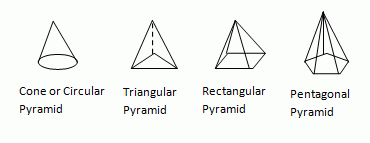Pyramids have different appearance depending on their base. Each segment of the base is elongated to form a triangle. If a pyramid is formed from an octagonal base, then 8 triangles are formed. In other words a pyramid is a polyhedron with a single base and lateral faces that are all triangular. All lateral edges of a pyramid meet at a single point, or vertex. Example:The base is a heptagon (7 sides) Answer: heptagonal pyramid Directions: Answer the following questions. Also draw at least five examples of pyramids on your own.
 Q 1: What is the name of this shape?octagonal pyramidtriangular pyramidheptagonal pyramid Q 2: What is the name of this shape?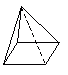rectangular pyramidtriangular pyramidpentagonal pyramid Q 3: What is the name of this shape?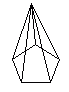rectangular pyramidpentagonal pyramidtriangular pyramid Q 4: What is the name of the shape?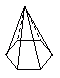triangular pyramidsquare pyramidhexagonal pyramid Q 5: What is the name of this shape?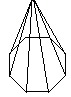pentagonal pyramidrectangular pyramidoctagonal pyramid Q 6: What is the name of this shape?rectangular pyramidpentagonal pyramidsquare pyramid Question 7: This question is available to subscribers only! Question 8: This question is available to subscribers only!